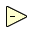# Subtract function

Object information
Owning palette(s) Numeric palette
Type Function
Requires Basic Development Environment
IconThe Subtract function computes the difference of the inputs.

If you wire two waveform values or two dynamic data type values to this function, error in and error out terminals appear on the function. Subtracting two time stamp values yields a numeric value (difference in time), and subtracting a numeric value from a time stamp value yields a time stamp. You cannot subtract a time stamp value from a numeric value. The dimensions of two matrices that you want to subtract must be the same. Otherwise, this function returns an empty matrix. The connector pane displays the default data types for this polymorphic function.

## Usage

When you wire matrix data as an input to this function, a VI that includes subVIs that work with the matrix data type replaces the function. The resulting VI has the same icon but contains a matrix-specific algorithm. The node remains a VI if you disconnect the matrix from the input(s). Wire other data types as inputs to restore the original function. If you wire a data type to a function and that data type causes a basic math operation to fail, the function returns an empty matrix or NaN.

### Fixed-Point

If you wire fixed-point values to Numeric functions such as Add, Subtract, Multiply, and Square, the functions usually return values that do not lose any bits of word length. However, if the operation creates a value that exceeds the maximum word length that LabVIEW accepts, overflow or rounding conditions can occur. LabVIEW accepts a maximum word length of 64 bits. Use the Numeric Node Properties dialog box to configure how LabVIEW handles overflow or rounding of fixed-point data.

## History

Version Change(s)More info to come.